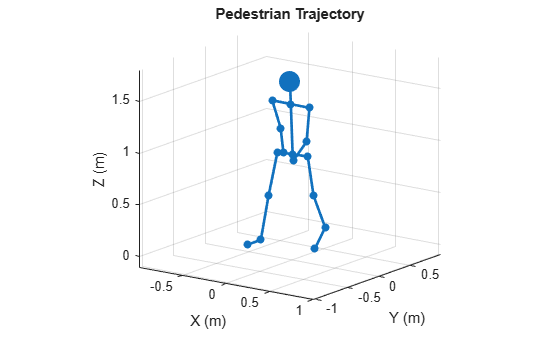# plot

Display stick figure showing the positions of all body segments of pedestrian

## Syntax

``plot(pedestrian)``
``fhndl = plot(pedestrian)``

## Description

example

````plot(pedestrian)` displays a stick figure showing the positions of all body segments of a pedestrian. The lines of the figure represent body segments while the dots represent the joints connecting body segments.```
````fhndl = plot(pedestrian)` returns the figure handle of the display window.```

## Examples

collapse all

Display the motion of a pedestrian walking a square path. Create the pedestrian using a `backscatterPedestrian` object with default values except for height which is 1.7 meters. Advance and display the pedestrian position every 3 milliseconds. First, the pedestrian moves along the positive x-axis, then along the positive y-axis, along the negative x-axis, and finally along the negative y-axis to return to the starting point.

```ped = backscatterPedestrian('Height',1.7); dt = 0.003; N = 3600; for m = 1:N if (m < N/4) angstep = 0.0; end if (m >= N/4) angstep = 90.0; end if (m >= N/2) angstep = 180.0; end if (m >= 3*N/4) angstep = 270.0; end move(ped,dt,angstep); plot(ped) end```## Input Arguments

collapse all

Pedestrian target, specified as a `backscatterPedestrian` object.

## Output Arguments

collapse all

Figure handle of plot window

### Topics

Introduced in R2019b